# Catalan Numbers

The Catalan numbers (1, 2, 5, 14, 42, 132, 429, 1430, 4862, 16796, 58786, 208012, 742900, 2674440, 9694845, ...), named after Eugène Charles Catalan (1814–1894), arise in a number of problems in combinatorics. They can be computed using this formula: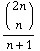Among other things, the Catalan numbers describe:

• the number of ways a polygon with n+2 sides can be cut into n triangles
• the number of ways to use n rectangles to tile a stairstep shape (1, 2, ..., n−1, n).
• the number of ways in which parentheses can be placed in a sequence of numbers to be multiplied, two at a time
• the number of planar binary trees with n+1 leaves
• the number of paths of length 2n through an n-by-n grid that do not fall below the main diagonal

The following figures show some of the interpretations—polygon divisions, binary trees, pairwise multiplication—combined. For n=2: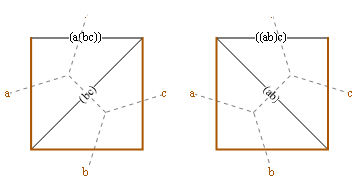And n=3: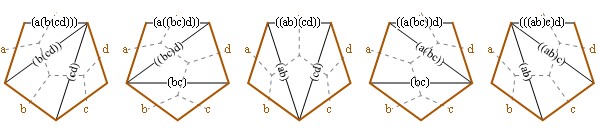Polygon diagrams:

4 sides, 2 ways: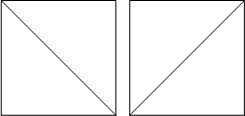5 sides, 5 ways: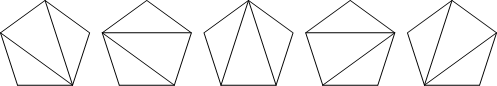6 sides, 14 ways: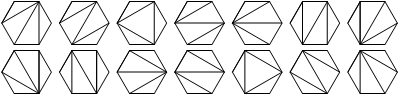7 sides, 42 ways: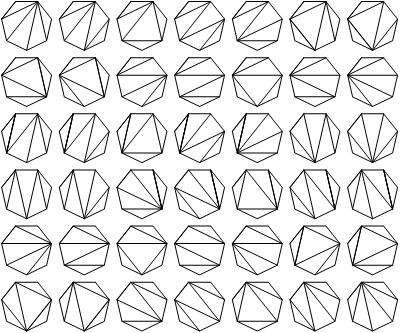8 sides, 132 ways: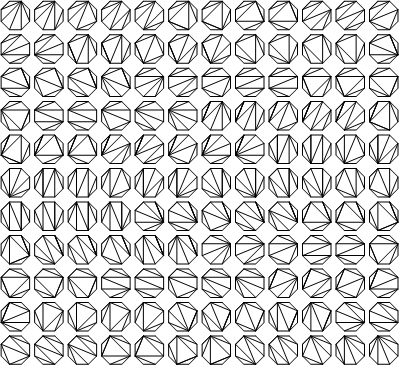9 sides, 429 ways:
(Hidden in file catalan9.png, as we used to do.)

Step diagrams:

2 rectangles, 2 ways:3 rectangles, 5 ways:4 rectangles, 14 ways: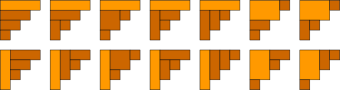5 rectangles, 42 ways: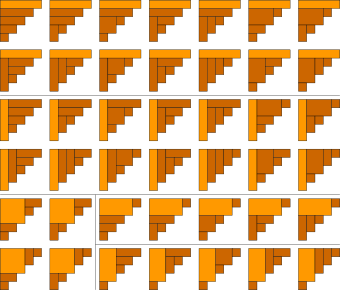6 rectangles, 132 ways: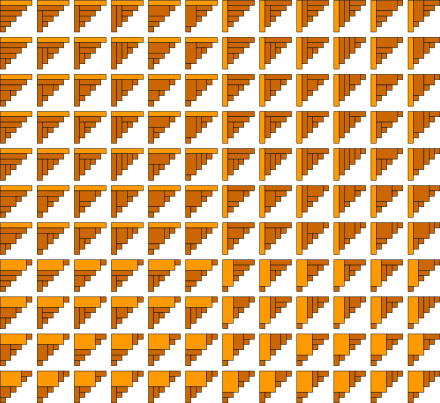Multiplication diagrams:

3 numbers:

`(1 (2 3))   ((1 2) 3)`

4 numbers:

```(1 (2 (3 4)))   (1 ((2 3) 4))
((1 2) (3 4))   ((1 (2 3)) 4)
(((1 2) 3) 4)```

5 numbers:

```(1 (2 (3 (4 5))))   (1 (2 ((3 4) 5)))
(1 ((2 3) (4 5)))   (1 ((2 (3 4)) 5))
(1 (((2 3) 4) 5))   ((1 2) (3 (4 5)))
((1 2) ((3 4) 5))   ((1 (2 3)) (4 5))
((1 (2 (3 4))) 5)   ((1 ((2 3) 4)) 5)
(((1 2) 3) (4 5))   (((1 2) (3 4)) 5)
(((1 (2 3)) 4) 5)   ((((1 2) 3) 4) 5)
```

6 numbers:

```(1 (2 (3 (4 (5 6)))))   (1 (2 (3 ((4 5) 6))))
(1 (2 ((3 4) (5 6))))   (1 (2 ((3 (4 5)) 6)))
(1 (2 (((3 4) 5) 6)))   (1 ((2 3) (4 (5 6))))
(1 ((2 3) ((4 5) 6)))   (1 ((2 (3 4)) (5 6)))
(1 ((2 (3 (4 5))) 6))   (1 ((2 ((3 4) 5)) 6))
(1 (((2 3) 4) (5 6)))   (1 (((2 3) (4 5)) 6))
(1 (((2 (3 4)) 5) 6))   (1 ((((2 3) 4) 5) 6))
((1 2) (3 (4 (5 6))))   ((1 2) (3 ((4 5) 6)))
((1 2) ((3 4) (5 6)))   ((1 2) ((3 (4 5)) 6))
((1 2) (((3 4) 5) 6))   ((1 (2 3)) (4 (5 6)))
((1 (2 3)) ((4 5) 6))   ((1 (2 (3 4))) (5 6))
((1 (2 (3 (4 5)))) 6)   ((1 (2 ((3 4) 5))) 6)
((1 ((2 3) 4)) (5 6))   ((1 ((2 3) (4 5))) 6)
((1 ((2 (3 4)) 5)) 6)   ((1 (((2 3) 4) 5)) 6)
(((1 2) 3) (4 (5 6)))   (((1 2) 3) ((4 5) 6))
(((1 2) (3 4)) (5 6))   (((1 2) (3 (4 5))) 6)
(((1 2) ((3 4) 5)) 6)   (((1 (2 3)) 4) (5 6))
(((1 (2 3)) (4 5)) 6)   (((1 (2 (3 4))) 5) 6)
(((1 ((2 3) 4)) 5) 6)   ((((1 2) 3) 4) (5 6))
((((1 2) 3) (4 5)) 6)   ((((1 2) (3 4)) 5) 6)
((((1 (2 3)) 4) 5) 6)   (((((1 2) 3) 4) 5) 6)
```

Tree diagrams:

3 nodes:4 nodes:5 nodes: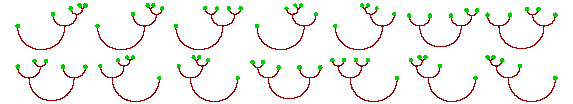6 nodes: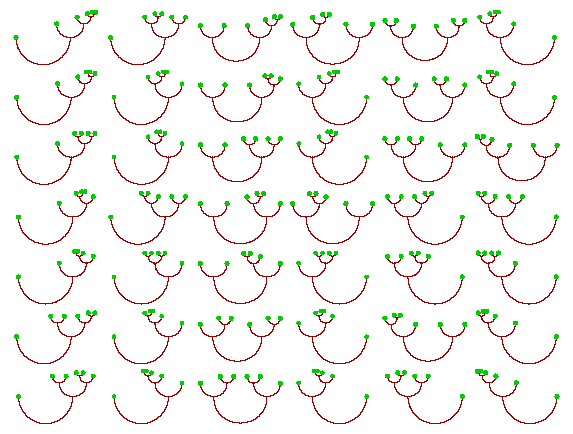Path diagrams:

2 × 2 grid: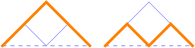3 × 3 grid: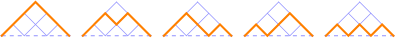4 × 4 grid: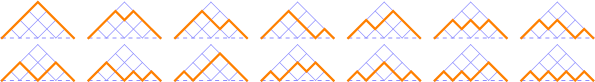5 × 5 grid: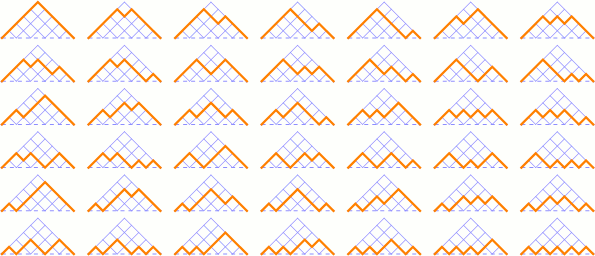The Catalan interpretations can be partially ordered and arranged into a lattice called a Tamari lattice: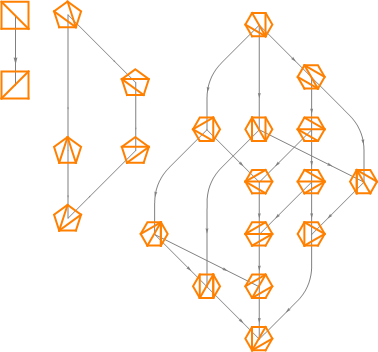You might also enjoy the generalization Fuss–Catalan numbers.

Originally designed and rendered using Mathematica 3.0 for the Apple Macintosh.

Inspiration and facts (though not figures) by Brian Hayes, A Question of Numbers [dead link], American Scientist, January–February 1996; Steven S. Skiena, Implementing Discrete Mathematics: Combinatorics and Graph Theory with Mathematica, Addison-Wesley, 1990; Fred S. Roberts, Applied Combinatorics, Prentice-Hall, 1984; D. E. Knuth, Sorting and Searching (vol. 3 of The Art of Computer Programming), Addison-Wesley, 1973. Catalan dates from Florian Cajori, A History of Mathematics, The Macmillan Company, 1922; R. P. Stanley, Enumerative Combinatorics, Volume 2, Cambridge: Cambridge University Press, 1999.

See also Martin Gardner, Time Travel and Other Mathematical Bewilderments, Chapter 20, W. H. Freeman, 1988; and Ilan Vardi, Computational Recreations in Mathematica, Chapter 9, Addison-Wesley, 1991.